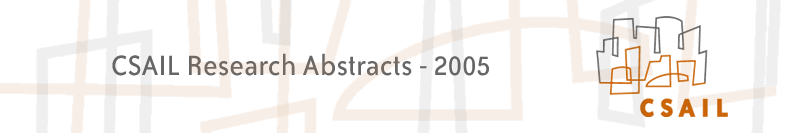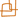Introduction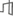Architecture, Systems & NetworksLanguage, Learning, Vision & GraphicsPhysical, Biological & Social Systems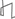Theory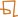### Automated Model Generation Using Qualitative Abstraction

#### Martin Sachenbacher and Brian C. Williams

##### Introduction

Simulating a system's behavior based on a model is a common task in many areas of engineering. On the other hand, research in Artificial Intelligence (AI) has come up with algorithms that exploit models for more advanced tasks such as diagnosis, planning, and reconfiguration. However, whereas engineering models for simulation are typically based on numerical (real-valued) differential equations, AI approaches for planning, diagnosis and reconfiguration are typically based on discrete (logical) models.  In this research effort, we aim to bridge this gap by developing techniques and tools to semi-automatically transform numerical simulation models (in particular, Matlab/Simulink models) to discrete (constraint-based) models. This automated modeling capability will further enhance our existing framework for model-based diagnosis, planning and reconfiguration 

##### Approach

Our approach is based on automatically abstracting numerical models by mapping the domains of continuous variables to small, discrete domains. Currently, it is assumed that these domain mappings are provided by the user. The application of a domain mapping to the variables of a continuous function turns the function into a discrete constraint (see Fig. 2). We have experimented with different techniques to abstract numerical equations given domain mappings. A first approach used interval arithmetic to locally abstract each operator in a numerical equation. However, the applicability of this approach to real-world engineering models turned out to be limited, because these models are often not represented in terms of analytic functions, but rather in terms of interpolated measurement points (characteristic maps). For instance, Fig. 1 shows part of a model of a gasoline engine, whose behavior is modeled as a combination of several characteristic maps. An additional complication is that simulation models currently used in industry tend to have several levels of hierarchy.

Based on these challenges, we developed a method for abstraction that can treat Matlab/Simulink functions as a "black box", and does not make assumptions about their internal structure. The basic idea is to approximate the relationship between the input and output variables in a function using stochastic sampling. This process is illustrated in Fig. 2. The domain mapping is then applied to the samples instead of the original function. By repeating the process with many samples, a numerical equation is approximated with a discrete constraint.  An advantage of this random sampling technique is that no assumptions need to be made about the functions, thus any function can be abstracted, including characteristic maps. However, random sampling suffers from the problem that the resulting constraint is only an approximation and tuples of the constraint might be missed because there is no sample in the respective region of the input space. This is also illustrated in Fig. 2. This incompleteness problem can be mitigated by increasing the number of samples in order to reduce the probability of missing tuples, or by exploiting properties of the function, such as monotonicity, in order to guarantee that the sampling is fair and no tuples are missed.

#####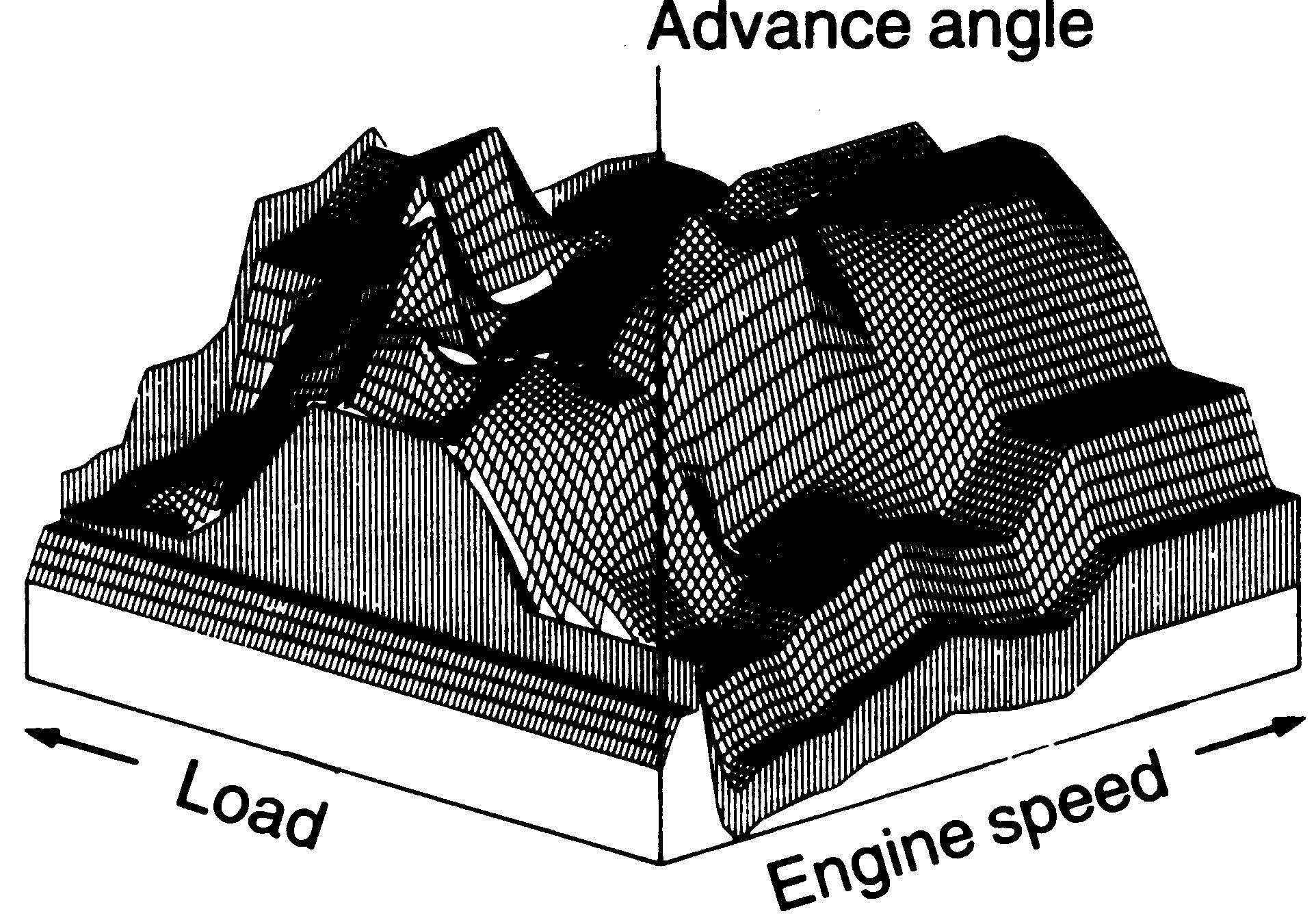Figure 1: Numerical model describing the behavior of a gasoline engine.

#####Figure 2: Function abstraction based on stochastic sampling. The grey boxes correspond to tuples of the resulting discrete constraint.

##### Progress

We applied the stochastic sampling method to a real-world Matlab/Simulink model from an automotive test-bed.  The model involved three sub-parts corresponding to a gasoline engine, a throttle, and an air intake manifold. For each of these sub-parts of the model, we attached their inputs to uniform random number generators to generate random input samples. The output of the Matlab/Simulink block for each input sample was then recorded as a Matlab data file. In order to ensure a good approximation of the functions, and thus minimize the probability of missed tuples, we generated between 100,000 and 500,000 samples for each function. The size of the resulting Matlab data files was between 2MB and 10MB.  We implemented a protoype of a model generator that maps these sampling points into discrete constraints as shown in Fig. 2. In addition, the prototype tries to compactly represent the resulting discrete constraints by encoding them symbolically in the form of decision diagrams . The obtained models can be fed into our framework for model-based diagnosis, planning and reconfiguration .  We have begun evaluating the automatically generated models for the task of model-based diagnosis using fast, discrete algorithms .

##### Future

We recently extended the approach from static equations to differential equations by generalizing from constraints to constraint automata. In order to further automate the generation of constraint-based models from numerical simulation models, we seek to automatically generate domain abstractions with the "right" (qualitative) granularity, instead of asking the user to provide them.  Our preliminary work in this direction is described in [2,3].  However, it seems likely that some parts of the model or the mapping still need to be defined manually, as the simulation model typically describes only the nominal behavior of components and does not contain information about the possible failures of components. The next steps in the project will be to further refine the model of the test-bed by including more components,  and to compare (for the case of diagnosis) our approach with hybrid estimation techniques that directly reason with the continuous model.

##### Research Support

This research is funded in part by the project "Model-based Programming, Self-Diagnosis and Repair", Toyota research agreement 5/1/04.

##### References

  Brian C. Williams. and Michel D. Ingham. Model-Based Programming: Controlling Embedded Systems by Reasoning About Hidden State, Proceedings of the International Conference on Principles and Practice of Constraint Programming (CP-02), Ithaca, New York, September 2002.

 Martin Sachenbacher and Peter Struss: Automated Qualitative Domain Abstraction. Proceedings of the 18th International Joint Conference on Artificial Intelligence (IJCAI-03), Acapulco, Mexico 2003.

 Martin Sachenbacher and Peter Struss: Task-dependent Qualitative Domain Abstraction. Artificial Intelligence 162 (1-2), 2005.

 Martin Sachenbacher and Brian C. Williams. Diagnosis as Semiring-based Constraint Optimization. Proceeedings of the 16th European Conference on Artificial Intelligence (ECAI'04), Valencia, Spain, 2004.

 R.I. Bahar, E.A. Frohm, C.M. Gaona, G.D. Hachtel, E. Macii, A. Pardo, and F. Somenzi. Algebraic Decision Diagrams and Their Applications. In IEEE /ACM International Conference on CAD, pages 188–191, Santa Clara, California, 1993.Computer Science and Artificial Intelligence Laboratory (CSAIL) The Stata Center, Building 32 - 32 Vassar Street - Cambridge, MA 02139 - USA tel:+1-617-253-0073 - publications@csail.mit.edu (Note: On July 1, 2003, the AI Lab and LCS merged to form CSAIL.)